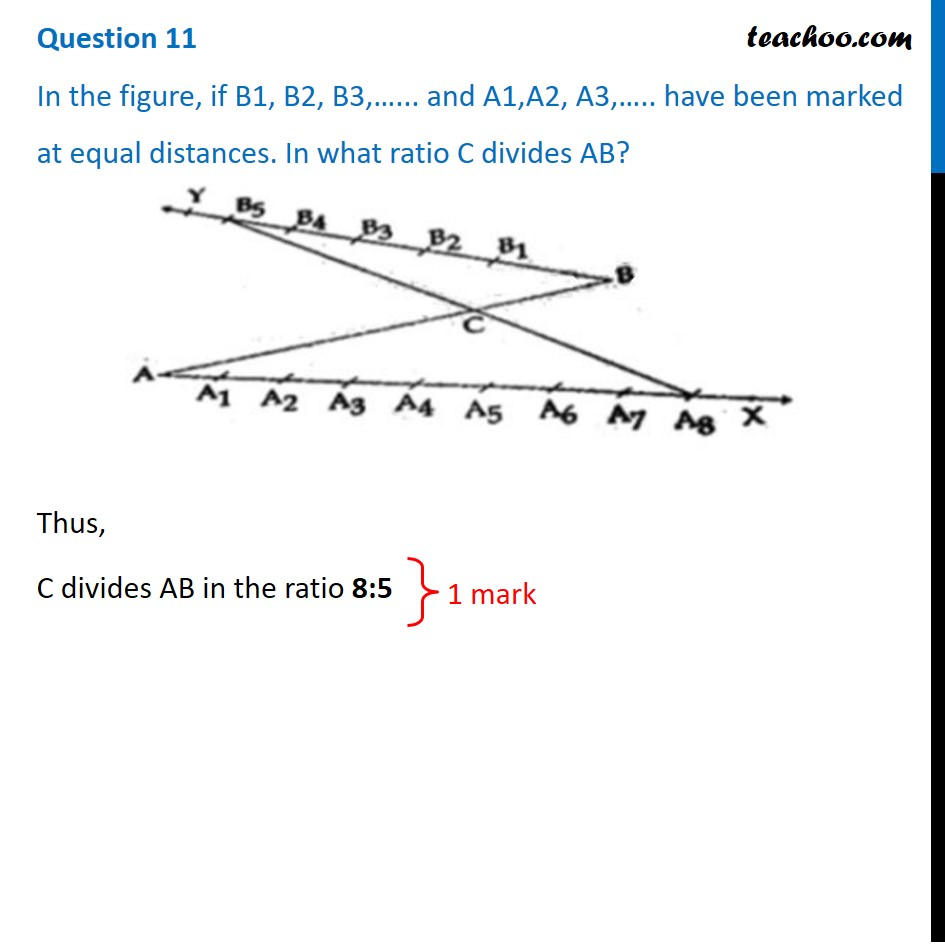## In the figure, if B1, B2, B3,…... and A1,A2, A3,….. have been marked at equal distances. In what ratio C divides AB?1. Class 10
2. Solutions of Sample Papers for Class 10 Boards
3. CBSE Class 10 Sample Paper for 2021 Boards - Maths Standard

Transcript

Question 11 In the figure, if B1, B2, B3,…... and A1,A2, A3,….. have been marked at equal distances. In what ratio C divides AB?Thus, C divides AB in the ratio 8:5

CBSE Class 10 Sample Paper for 2021 Boards - Maths Standard

Class 10
Solutions of Sample Papers for Class 10 Boards